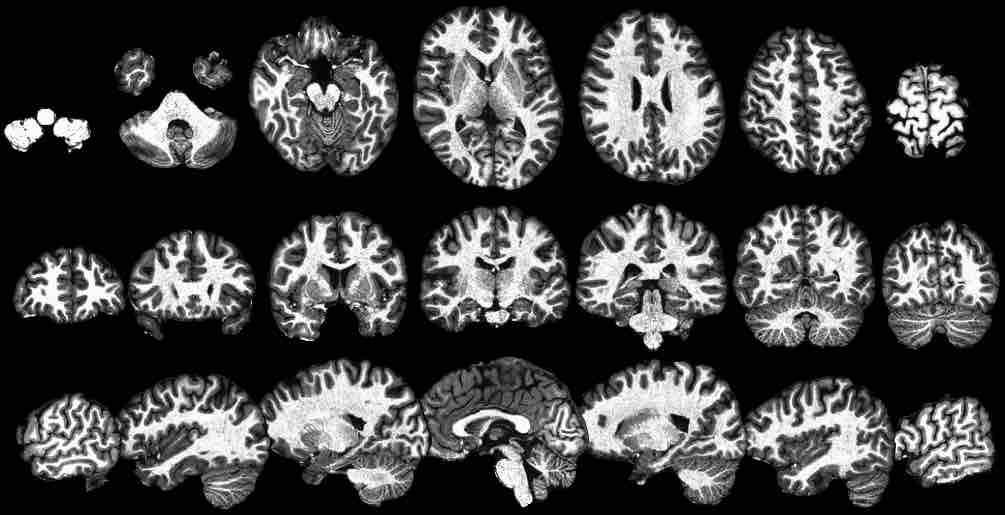# Vector similarity reference

This is a quick post to illustrate with python code how several common vector similarity computations are related to each other. For more details I highly encourage you to check out Brendan O’Connor’s really nice elaboration.

# Import some stuff
import numpy as np
import pandas as pd
import scipy.spatial.distance as spd
from pymer4.simulate import easy_multivariate_normal
from pymer4.models import Lm

# Simulate some data
# 2, 50 dimensional vectors correlated ~ r = -0.05
X = easy_multivariate_normal(50,2,corrs=-.05)
a, b = X[:,0], X[:,1]


## Inner product

Sum of element wise multiplication

$\sum_i {x_iy_i} = x \cdot y$
np.dot(a,b)
-2.8071010155482368


## Covariance

Average centered inner product

$\frac{(x - \bar x) \cdot (y - \bar y)}{n}$
a_centered = a - a.mean()
b_centered = b - b.mean()

np.dot(a_centered,b_centered) / len(a)
-0.06920945007922867

# Check our work
np.cov(a,b,ddof=0)
-0.06920945007922867


## Cosine Similarity

Normalized (L2) inner product

$\frac{x \cdot y}{||x|| \ ||y||}$
# Euclidean/L2 norm = square root of sum of squared values
# algebra form
a_norm = np.sqrt(np.sum(np.power(a,2)))
# matrix form; transpose is not strictly needed here, just for illustration
b_norm = np.sqrt(np.dot(b,b.T))

# numpy short-cut: np.linalg.norm(a)
np.dot(a,b) / (a_norm * b_norm)
-0.049727707335311774

# Check our work (subtract 1 because scipy returns distances)
1 - spd.cosine(a,b)
-0.04972770733531173


## Pearson Correlation

Centered, normalized (L2) inner product

$\frac{(x - \bar x) \cdot (y - \bar y)}{||x - \bar x|| \ ||y - \bar y||}$
# Can think of this as normalized covariance OR centered cosine similarity
a_centered_norm = np.linalg.norm(a_centered)
b_centered_norm = np.linalg.norm(b_centered)
np.dot(a_centered,b_centered) / (a_centered_norm * b_centered_norm)
-0.06299972596364047

# Check our work
1 - spd.correlation(a,b)
-0.06299972596364034


## OLS (univariate w/o intercept)

Partially normalized inner product, where partially means using a single vector’s norm

$\frac{x \cdot y}{||x||^2}$
# Can think of this as cosine similarity using only one vector's norm
np.dot(a,b) / (a_norm * a_norm)
-0.06164964567554537

# Check our work against a regression in pymer4
model = Lm('B ~ 0 + A',data=pd.DataFrame({'A':a,'B':b}))
model.fit(summarize=False)
# Grab the beta value directly from the coefficients table.
model.coefs.iloc[-1,0]
-0.06164964567554537


## OLS (univariate w/ intercept)

Centered, partially normalized inner product

$\frac{(x - \bar x) \cdot y}{||x - \bar x||^2}$
# In the numerator we could actually center a or b, or both.
np.dot(a_centered,b) / (a_centered_norm * a_centered_norm)
-0.07620054357106859

# Check our work
model = Lm('B ~ A',data=pd.DataFrame({'A':a,'B':b}))
model.fit(summarize=False)
model.coefs.iloc[-1,0]
-0.07620054357106855